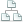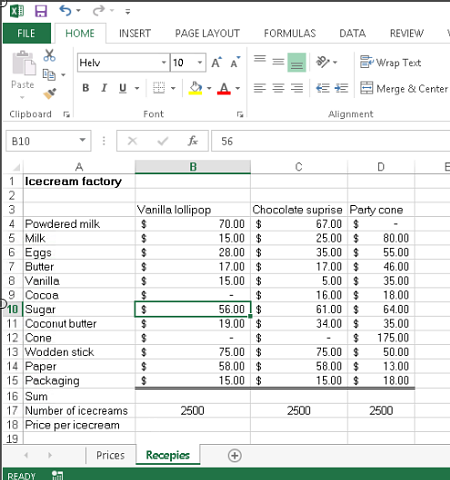Home >Tips >Excel formulas between sheetsSite map

# Excel Formulas Between Sheets

## An Excel tutorial by Peter KalmstromThe possibility to tell Excel to calculate is a crucial feature and the most important reason to use it. In other demos Peter Kalmstrom has shown how to create formulas for plus and minus and how to make Excel multiply and divide.

When you use a formula, Excel calculates with the values of the cells you have specified in the formula and gives the result in a separate cell. When the values in the cells are changed, the figure in the result cell will change automatically.

In the demo below Peter shows how you can take values for the calculation from another Excel sheet than the one where you want the result to be displayed.

As an example Peter uses calculations on the prices for three different kinds of ice cream. He has the ice cream ingredients and their costs on one Excel sheet, and he wants the cost per ice cream to go into another sheet where he calculates what price to take for each kind of ice cream.Peter uses Excel 2013 for his demo, but the formulas are the same for earlier versions of Excel.

You might also be interested in studying the Tip about the Excel lookup and data validation functions.

Follow kalmstrom.com on LinkedInFacebook or Twitter!

 Products Buy FAQ Services Tips Books Contact About Us Tools
 Security and integrity Copyright  Kalmstrom Enterprises AB  All rights reserved